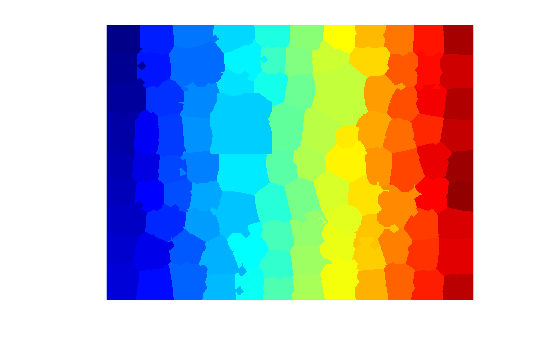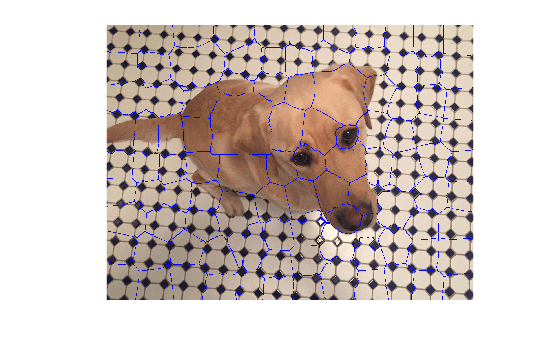Find region boundaries of segmentation

Description

example

mask = boundarymask(L) computes a mask that represents the region boundaries for the input label matrix L. The output, mask, is a logical image that is true at boundary locations and false at non-boundary locations.

mask = boundarymask(BW) computes the region boundaries for the input binary image BW.

mask = boundarymask(___,conn) computes the region boundaries using a connectivity specified by conn.

Examples

collapse all

Read an image into the workspace.

Create a superpixel representation of the image, returned as a label matrix.

L = superpixels(I,100);

Display the label matrix.

imshow(label2rgb(L))Find the region boundaries of the label matrix.

Display the boundary mask over the original image by using the labeloverlay function. The region boundaries of the label matrix appear as 1-pixel wide cyan lines.Input Arguments

collapse all

Label matrix, specified as a 2-D numeric matrix of nonnegative numbers or a 2-D logical matrix.

Data Types: single | double | int8 | int16 | int32 | uint8 | uint16 | uint32 | logical

Binary image, specified as a numeric or logical matrix of the same size as L.

Data Types: single | double | int8 | int16 | int32 | int64 | uint8 | uint16 | uint32 | logical

Pixel connectivity, specified as 4 or 8.

Value

Meaning

Two-Dimensional Connectivities

4-connected

Pixels are connected if their edges touch. The neighborhood of a pixel are the adjacent pixels in the horizontal or vertical direction.8-connected

Pixels are connected if their edges or corners touch. The neighborhood of a pixel are the adjacent pixels in the horizontal, vertical, or diagonal direction.Data Types: single | double | int8 | int16 | int32 | uint8 | uint16 | uint32

Output Arguments

collapse all

Rasterized grid of region boundaries, specified as a 2-D logical matrix of the same size as the input image. A pixel in mask is true when the corresponding pixel in the input image with value P has a neighboring pixel with a different value than P.

Data Types: logical